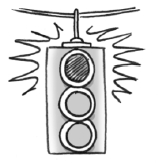### Home > A2C > Chapter 1 > Lesson 1.1.2 > Problem1-26

1-26.

Find the error in the solution below. Explain what the error is and solve the equation correctly. Show how to check your solution to be sure that it is correct.

$3(x−2)−2(x+7)=2x+17$
$3x−6−2x+14=2x+17$
$x+8=2x+17$
$−9=x$Note: The stoplight icon to the right of a problem indicates that there is an error in the problem.

Notice that the binomial $(x+7)$ is being multiplied by $−2$.

Use the distributive property correctly and continue solving.

The error is in line $2$.
It should be $−14$ not $+14$.
$3x−6−2x−14=2x+17$
Continue solving to get $x=−37$.

Substitute $−37$ for $x$ in the original equation and simplify both sides to compare results.

Result should be $−57=-57$.